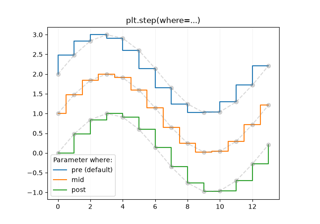# matplotlib.axes.Axes.step¶

Axes.step(self, x, y, *args, where='pre', data=None, **kwargs)[source]

Make a step plot.

Call signatures:

step(x, y, [fmt], *, data=None, where='pre', **kwargs)
step(x, y, [fmt], x2, y2, [fmt2], ..., *, where='pre', **kwargs)


This is just a thin wrapper around plot which changes some formatting options. Most of the concepts and parameters of plot can be used here as well.

Note

This method uses a standard plot with a step drawstyle: The x values are the reference positions and steps extend left/right/both directions depending on where.

For the common case where you know the values and edges of the steps, use stairs instead.

Parameters: xarray-like1D sequence of x positions. It is assumed, but not checked, that it is uniformly increasing. yarray-like1D sequence of y levels. fmtstr, optionalA format string, e.g. 'g' for a green line. See plot for a more detailed description. Note: While full format strings are accepted, it is recommended to only specify the color. Line styles are currently ignored (use the keyword argument linestyle instead). Markers are accepted and plotted on the given positions, however, this is a rarely needed feature for step plots. dataindexable object, optionalAn object with labelled data. If given, provide the label names to plot in x and y. where{'pre', 'post', 'mid'}, default: 'pre'Define where the steps should be placed: 'pre': The y value is continued constantly to the left from every x position, i.e. the interval (x[i-1], x[i]] has the value y[i]. 'post': The y value is continued constantly to the right from every x position, i.e. the interval [x[i], x[i+1]) has the value y[i]. 'mid': Steps occur half-way between the x positions. list of Line2DObjects representing the plotted data. **kwargsAdditional parameters are the same as those for plot.

Notes

## Examples using matplotlib.axes.Axes.step¶